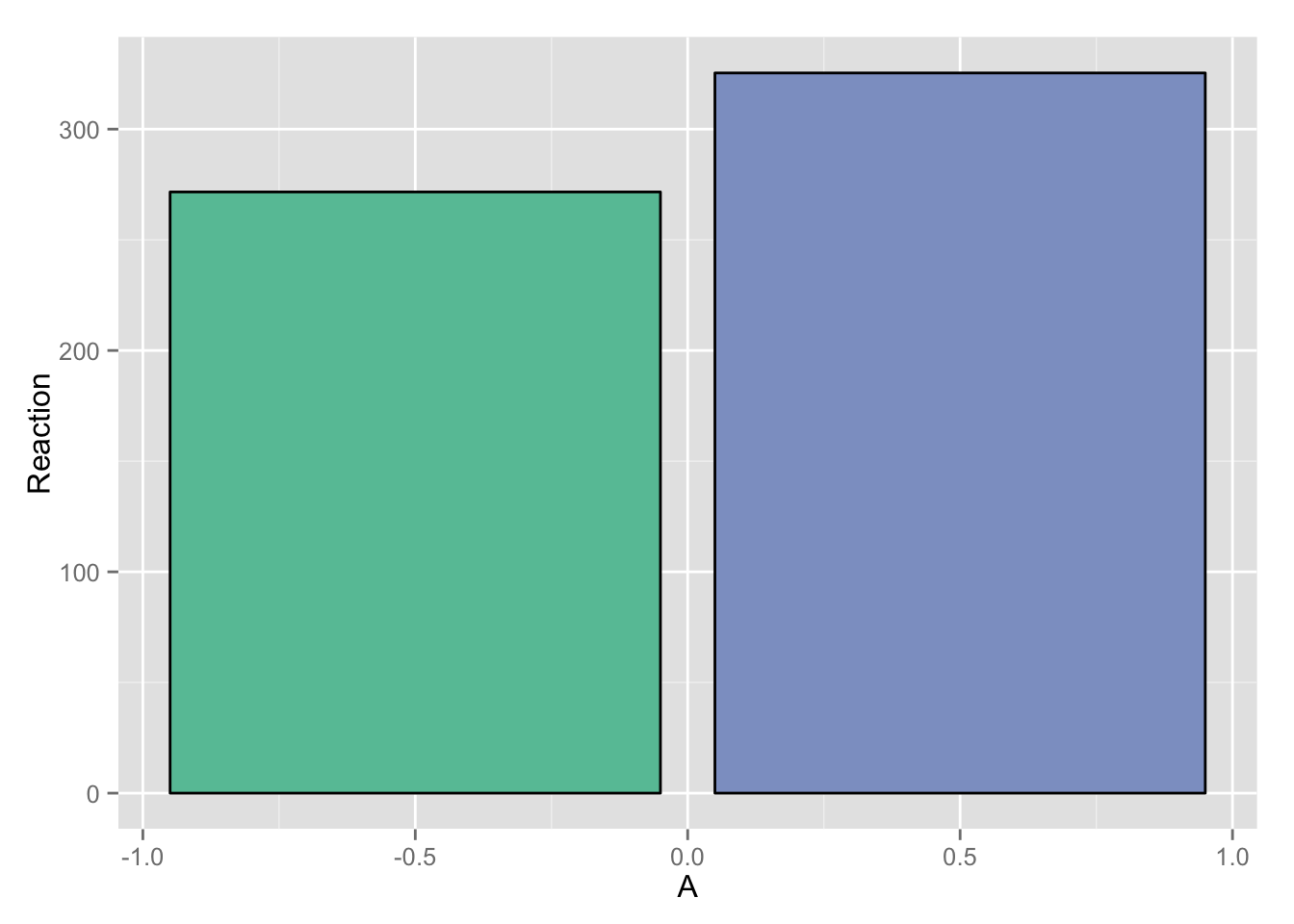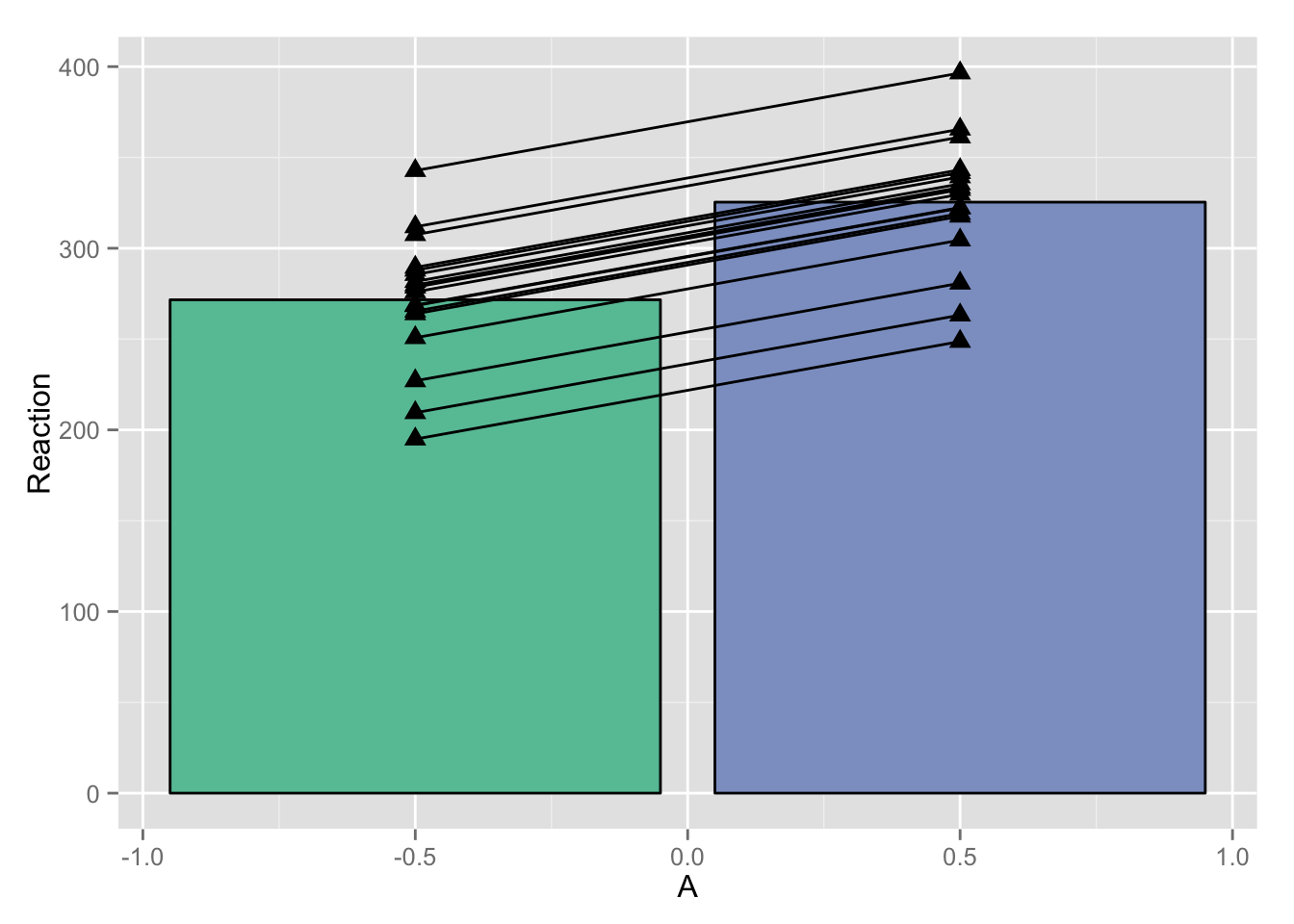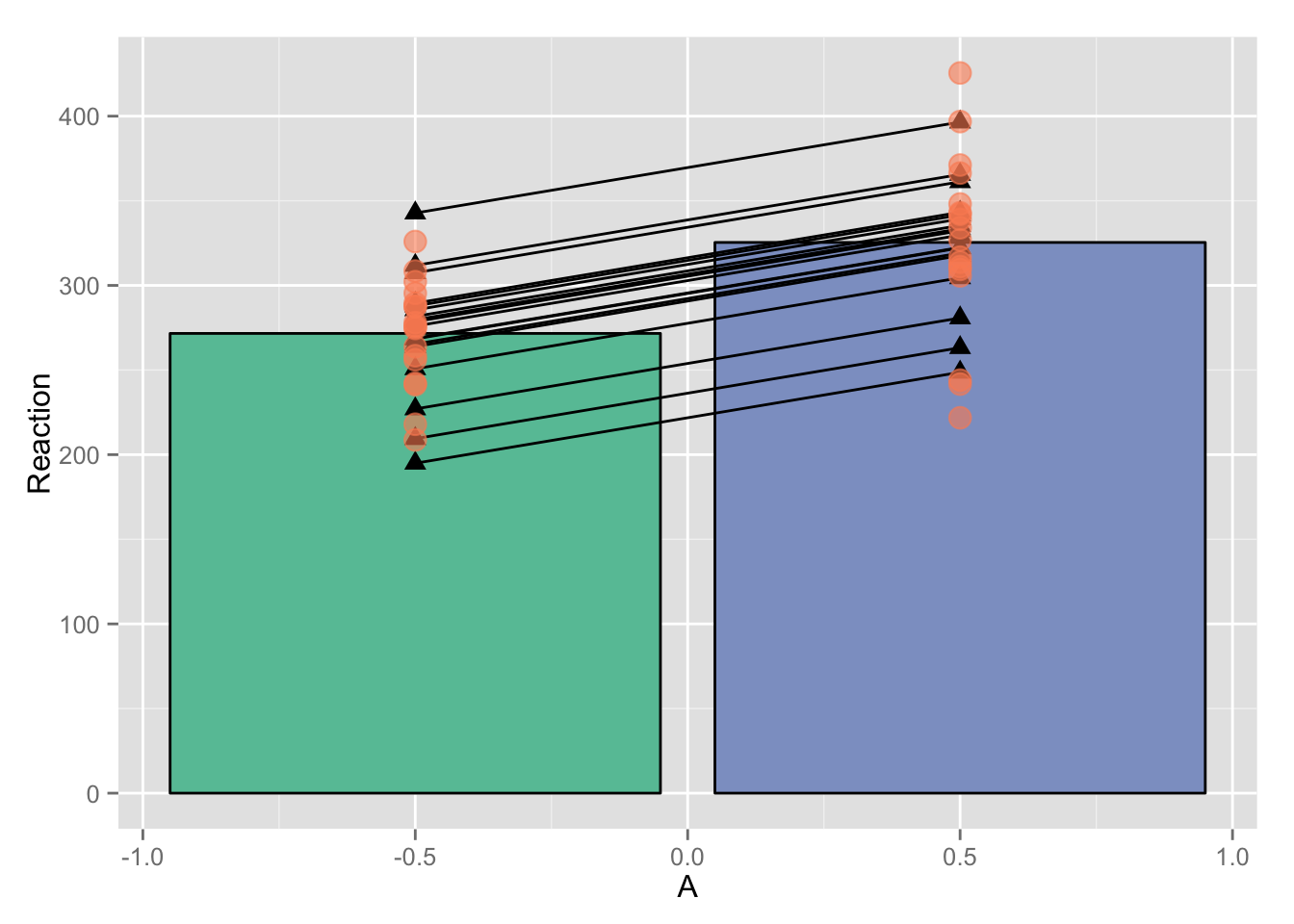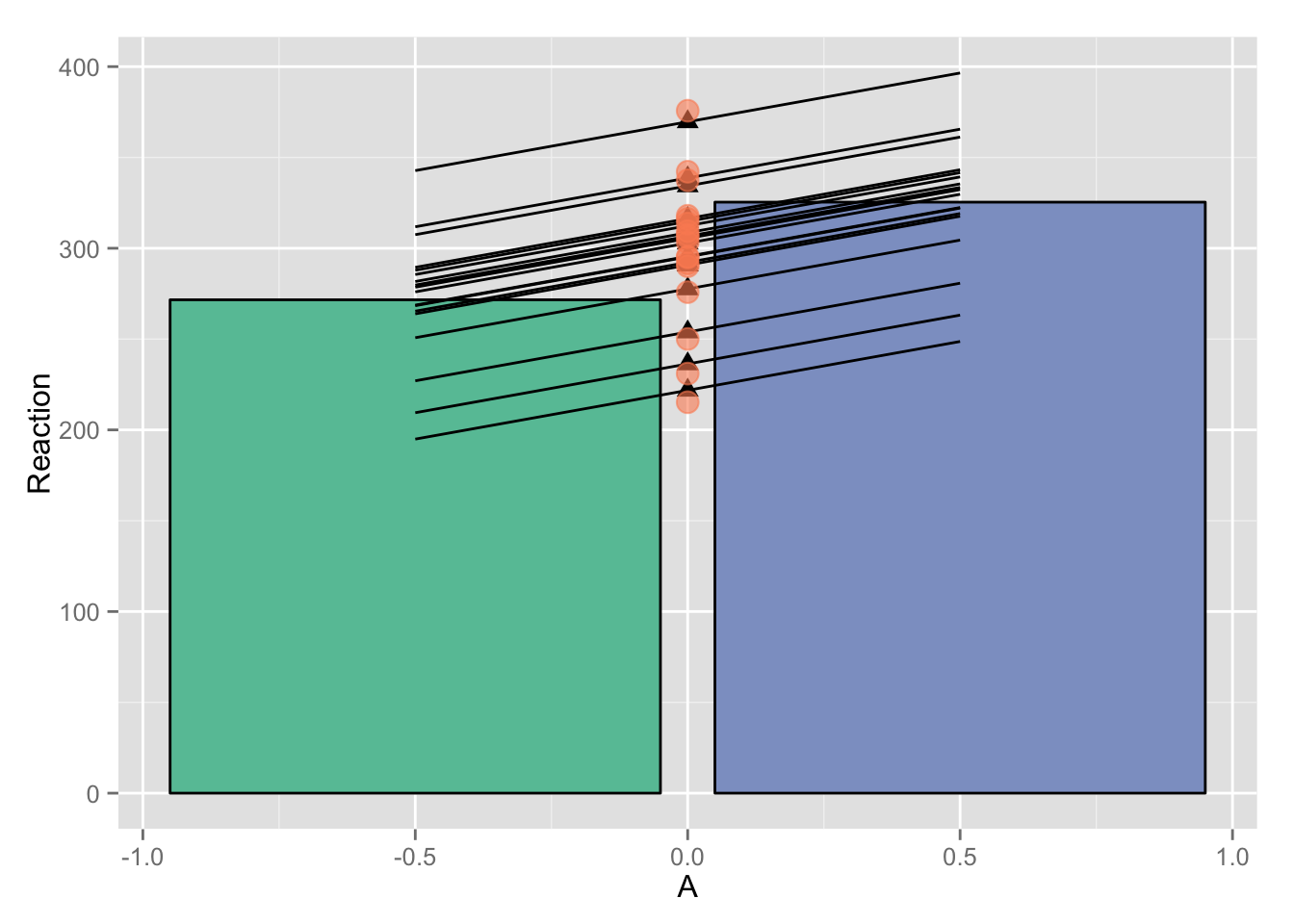I’ll be exploring the differences between these three models:

``````subj_intercepts_mod <- lmer(rt ~ A + (1|Subject))
subjA_intercepts_mod <- lmer(rt ~ 1 + (1|Subject:A))
subj_slopes_mod <- lmer(rt ~ A + (A|Subject))``````

Granted, the second model is rarely encountered in practice. More common would be a nested structure (see this crossvalidated post).

``````lmer(rt ~ 1 + (1|Subject) + (1|Subject:A))
## the above random effects structure is often written as `(1|Subject/A)` the
## same way `y ~ A + B + A:B` is usually written as `y ~ A * B`.``````

Even though the non-nested `(1|Subject:A)` situation is rare, I still find it informative as an example when exploring these models.

I’ll work with a modified version of the sleepstudy dataset from `lme4`.

``````library(lme4)
library(ggplot2)

library(dplyr)
library(reshape2)
library(broom)    # install_github("dgrtwo/broom")

library(stringr)

example <- sleepstudy %>%
mutate(A = ifelse(Days < 5, -0.5, 0.5)) %>%
select(Subject, A, Reaction)
head(example, n = 11)``````
``````##    Subject    A Reaction
## 1      308 -0.5    249.6
## 2      308 -0.5    258.7
## 3      308 -0.5    250.8
## 4      308 -0.5    321.4
## 5      308 -0.5    356.9
## 6      308  0.5    414.7
## 7      308  0.5    382.2
## 8      308  0.5    290.1
## 9      308  0.5    430.6
## 10     308  0.5    466.4
## 11     309 -0.5    222.7``````

I’ll start by plotting the means.

``````base_plot <- ggplot(example, aes(x = A, y = Reaction)) +
stat_summary(aes(fill = factor(A)), fun.y = mean, geom = "bar", color = "black") +
scale_fill_manual(values = c("#66c2a5", "#8da0cb")) +  # colorbrewer2.org
theme(legend.position = "none")
base_plot``````# Part 1: `subj_intercepts_mod`

First I’ll fit an `lmer` model that allows the intercept to vary across subjects.

``````subj_intercepts_mod <- lmer(Reaction ~ A + (1|Subject), data = example)
# broom::tidy turns `fixef(subj_intercepts_mod)` into a data.frame
fixed_params <- tidy(subj_intercepts_mod, effects = "fixed")[,c("term", "estimate")]
fixed_params``````
``````##          term estimate
## 1 (Intercept)   298.51
## 2           A    53.76``````

Although it’s sometimes helpful to think about model parameters (and you can draw them easily with `ggplot2::geom_abline`), I find it more beneficial in a simple design like this to deal in estimates. I’ll write a little function to speed up this conversion that seems like overkill now but it will come in handy later.

``````# converts parameters of a `Reaction ~ (Intercept) + A` model into estimates,
# assumes A is a 0-centered, unit-weighted, dichotomous variable
convert_parameters_to_estimates <- function(tidy_frame, id_var = ".") {
tidy_frame %>%
dcast(as.formula(paste(id_var, "term", sep="~")), value.var = "estimate") %>%
mutate(`-0.5` = `(Intercept)` - A/2, `0.5` = `(Intercept)` + A/2) %>%
select(-`(Intercept)`, -A) %>%
melt(idvars = id_var, measure.vars = c("-0.5", "0.5"),
variable.name = "A", value.name = "Reaction") %>%
mutate(A = as.numeric(as.character(A)))
}

fixed_estimates <- convert_parameters_to_estimates(fixed_params)[,c("A", "Reaction")]
fixed_estimates``````
``````##      A Reaction
## 1 -0.5    271.6
## 2  0.5    325.4``````
``````# sanity check
example %>% group_by(A) %>% summarize(Reaction = mean(Reaction)) %>%
merge(., fixed_estimates, by = "A", suffixes = c("_mean", "_model"))``````
``````##      A Reaction_mean Reaction_model
## 1 -0.5         271.6          271.6
## 2  0.5         325.4          325.4``````

It’s possible to turn parameters from the model into estimates that make sense; now let’s do the same thing with random effects. How will the model’s random effect parameters, when converted to estimates, compare to the averages for each subject that we can calculate by hand?

``````random_params <- tidy(subj_intercepts_mod, effect = "random")
random_estimates <- convert_parameters_to_estimates(random_params, id_var = "level")

fixed_slopes_plot <- base_plot +
geom_point(data = random_estimates, shape = 17, size = 3) +
geom_line(aes(group = level), data = random_estimates)
fixed_slopes_plot````````````fixed_slopes_plot +
stat_summary(aes(group = Subject), fun.y = mean,  # means from raw data
geom = "point", shape = 19, size = 4, color = "#fc8d62", alpha = 0.6)``````Of course the reason the two sets of points don’t line up is because we are only allowing the subject’s overall Reaction to vary, not the subject’s overall Reaction in each condition. Applying the same slope to each subject, this is the best we can do to account for variance:

``````base_plot +
geom_line(aes(group = level), data = random_estimates) +
## calculate mean Reaction by subject using `stat_summary`
stat_summary(aes(x=0.0, y=Reaction, group=level), data = random_estimates, fun.y = mean,
geom = "point", shape = 17, size = 3) +
stat_summary(aes(x=0.0, group=Subject), fun.y = mean,
geom = "point", shape = 19, size = 4, color = "#fc8d62", alpha = 0.6)````````````## Well, **almost** accurate estimates of each subject's overall mean Reaction.
## Note that the random estimate means (triangles) are closer to the overall mean,
## reflecting that the model assumes each subject's mean is closer to the overall
## average than it actually is---a fundamental "assumption" of a multilevel model.``````

## Conclusion

``subj_intercepts_mod <- lmer(Reaction ~ A + (1|Subject))``

A model that allows intercepts to vary across subjects does just that: it does a great job of estimating overall Reaction for each subject, but it is limited in estimating the effect of `A` on Reaction.

# Part 2: `subjA_intercepts_mod`

We’re looking for a way to capture the fact that all of the following by-subject lines don’t have the same slope.

``````subj_means_plot <- base_plot +
stat_summary(aes(group = Subject), fun.y = mean,  # means from raw data
geom = "point", shape = 19, size = 4, color = "#fc8d62") +
stat_summary(aes(group = Subject), fun.y = mean,
geom = "line", size = 1.2, color = "#fc8d62")
subj_means_plot``````